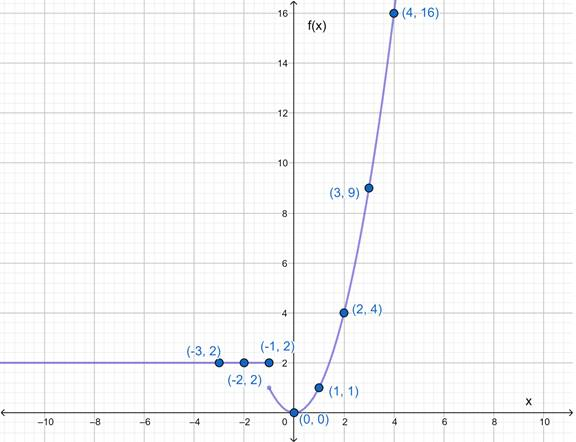# The graph of the given function.### Precalculus: Mathematics for Calcu...

6th Edition
Stewart + 5 others
Publisher: Cengage Learning
ISBN: 9780840068071### Precalculus: Mathematics for Calcu...

6th Edition
Stewart + 5 others
Publisher: Cengage Learning
ISBN: 9780840068071

#### Solutions

Chapter 2.2, Problem 41E
To determine

## To sketch : The graph of the given function.

Expert Solution

### Explanation of Solution

Given information :

f(x)={2      if  x1x2    if  x>1

Graph :

 x −3 −2 −1 0 1 2 3 4 f(x) 2 2 2 0 1 4 9 16This is the graph of the given piecewise defined equation.

Interpretation : The part of the graph to the left of x=1 coincides with the graph of f(x)=2 and the part of the graph to the right of x=1 coincides with the graph of f(x)=x2 . The open dot at (1,1) indicates that this point is excluded from the graph.

### Have a homework question?

Subscribe to bartleby learn! Ask subject matter experts 30 homework questions each month. Plus, you’ll have access to millions of step-by-step textbook answers!